Like   Tweet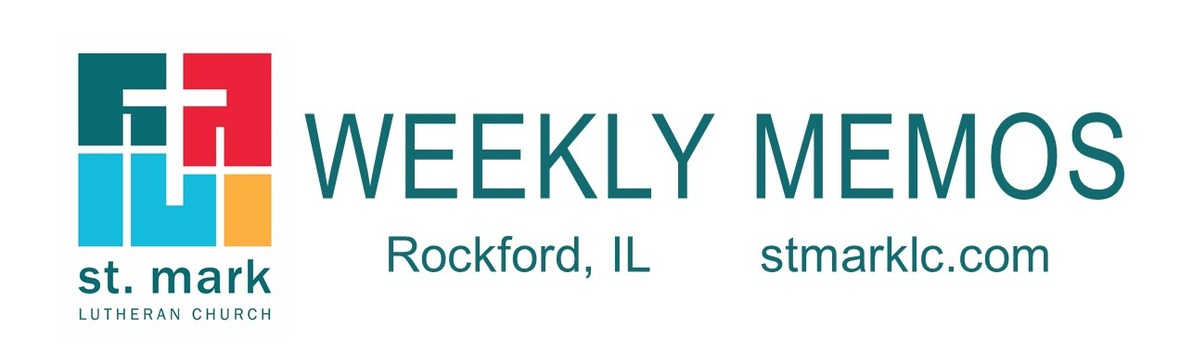## Pastoral Reflection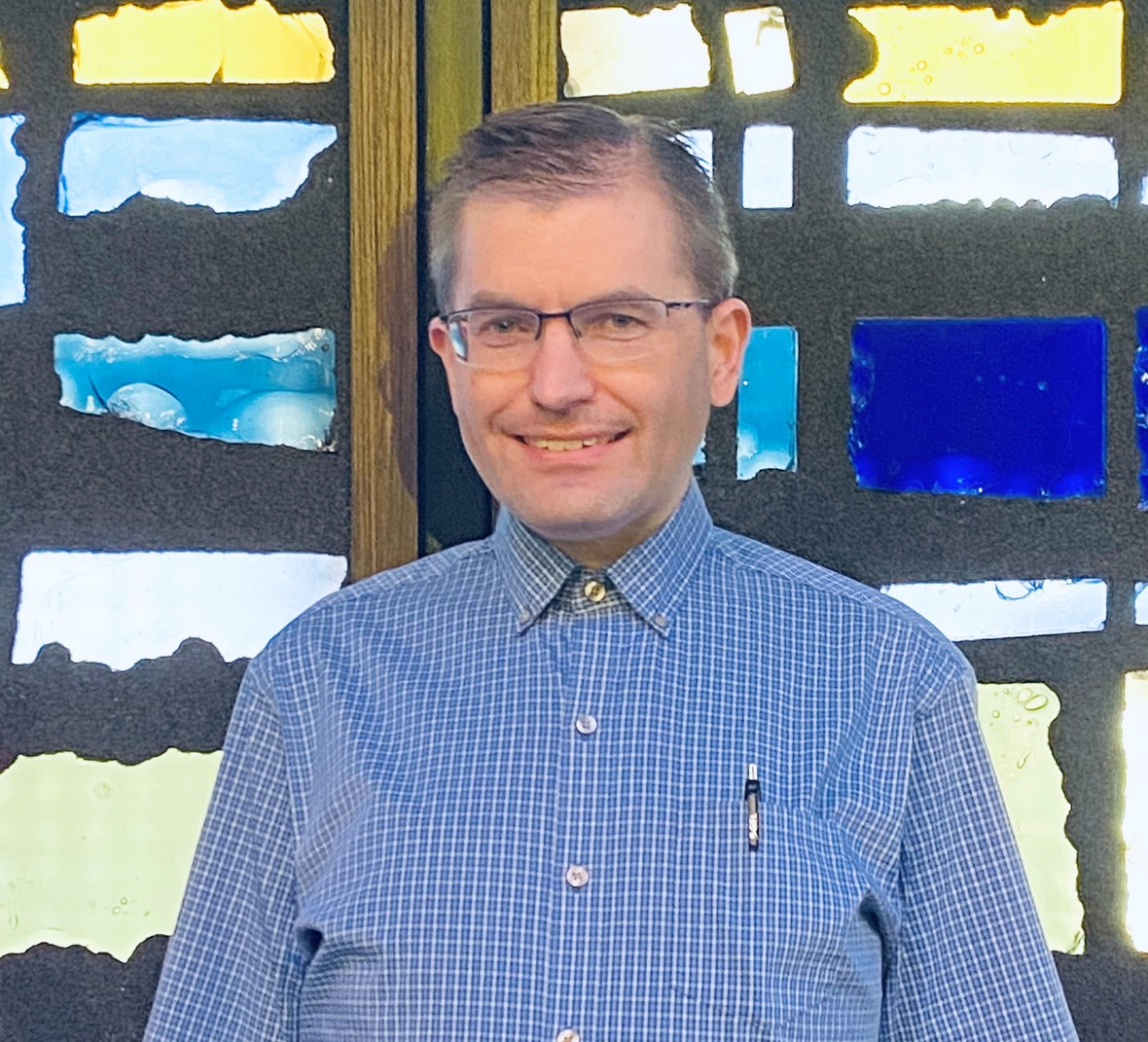Service of Lament and Observance of
Christ the King

Pastor Robert Franek

As co-chair of the Northern Illinois Synod Worship Committee, I have the privilege of working with a dedicated team in planning worship for our life-together as people and congregations of the Northern Illinois Synod. In collaboration with our synod staff, we are pleased to offer this Service of Lament and Observance of Christ the King. Read more.

 table div table+table+table+table div table{width:100%;padding:0}table div table+table+table+table div table img{width:96.23%;padding:0;float:none}table div table+table+table+table div table td{width:100%;padding:0 1.88% 18px}/* styles */## Sunday, November 22

9:30 A.M. Drive-In Outdoor Worship Service
Congregational Meeting immediately following
11:00 A.M. Remote Sunday School

## Tuesday, November 24

10:00 A.M. Staff Meeting
1:30 - 7:00 P.M. Blood Drive in Fellowship Hall
7:00 P.M. Council Meeting

## Wednesday, November 25

9:30 A.M. St. Mark Ringers
6:30 P.M. Recorded Thanksgiving Eve Worship Service available on our YouTube channel

## Thursday, November 26

Happy Thanksgiving!

Office Closed

## Sunday, November 29 First Sunday of Advent

9:30 A.M. Live-Stream Worship Service
11:00 A.M. Remote Sunday School

 table div table+table+table+table+table+table+table+table div table{width:100%;padding:0}table div table+table+table+table+table+table+table+table div table img{width:96.23%;padding:0;float:none}table div table+table+table+table+table+table+table+table div table td{width:100%;padding:0 1.88% 18px}/* styles */## News and Events

 table div table+table+table+table+table+table+table+table+table+table div table{width:100%;padding:0}table div table+table+table+table+table+table+table+table+table+table div table img{width:96.23%;padding:0;float:none}table div table+table+table+table+table+table+table+table+table+table div table td{width:100%;padding:0 1.88% 18px}/* styles */THIS SUNDAY: OUTDOOR WORSHIP & CONGREGATIONAL MEETING AT 9:30 A.M.

 table div table+table+table+table+table+table+table+table+table+table+table+table div table{width:100%;padding:0}table div table+table+table+table+table+table+table+table+table+table+table+table div table img{width:96.23%;padding:0;float:none}table div table+table+table+table+table+table+table+table+table+table+table+table div table td{width:100%;padding:0 1.88% 18px}/* styles */Due to the most recent round of Covid mitigations, all in-person gatherings at St. Mark must be put on hold.
This includes Bible studies, book clubs, circles, committee meetings, and Confirmation. Small groups are encouraged to meet remotely; please contact the church office for assistance. At this time, upcoming outdoor events, including Sunday’s service and congregational meeting, will still occur. The blood drive on November 24th will occur with added safety measures. Please contact the church office if you have any questions. You can read more about the newest mitigations on the state’s website: https://coronavirus.illinois.gov/s/restore-illinois-mitigation-plan

 table div table+table+table+table+table+table+table+table+table+table+table+table+table+table div table{width:100%;padding:0}table div table+table+table+table+table+table+table+table+table+table+table+table+table+table div table img{width:96.23%;padding:0;float:none}table div table+table+table+table+table+table+table+table+table+table+table+table+table+table div table td{width:100%;padding:0 1.88% 18px}/* styles *//* styles */ Home Communion Kits are available during office hours Monday - Friday 9:00 - 1:00 P.M. If you need to come by after these hours, please call the church office at 815-398-3557. Watch this short video from Pastor Chad or read more here.
 table div table+table+table+table+table+table+table+table+table+table+table+table+table+table+table+table div table{width:100%;padding:0}table div table+table+table+table+table+table+table+table+table+table+table+table+table+table+table+table div table img{width:96.23%;padding:0;float:none}table div table+table+table+table+table+table+table+table+table+table+table+table+table+table+table+table div table td{width:100%;padding:0 1.88% 18px}/* styles */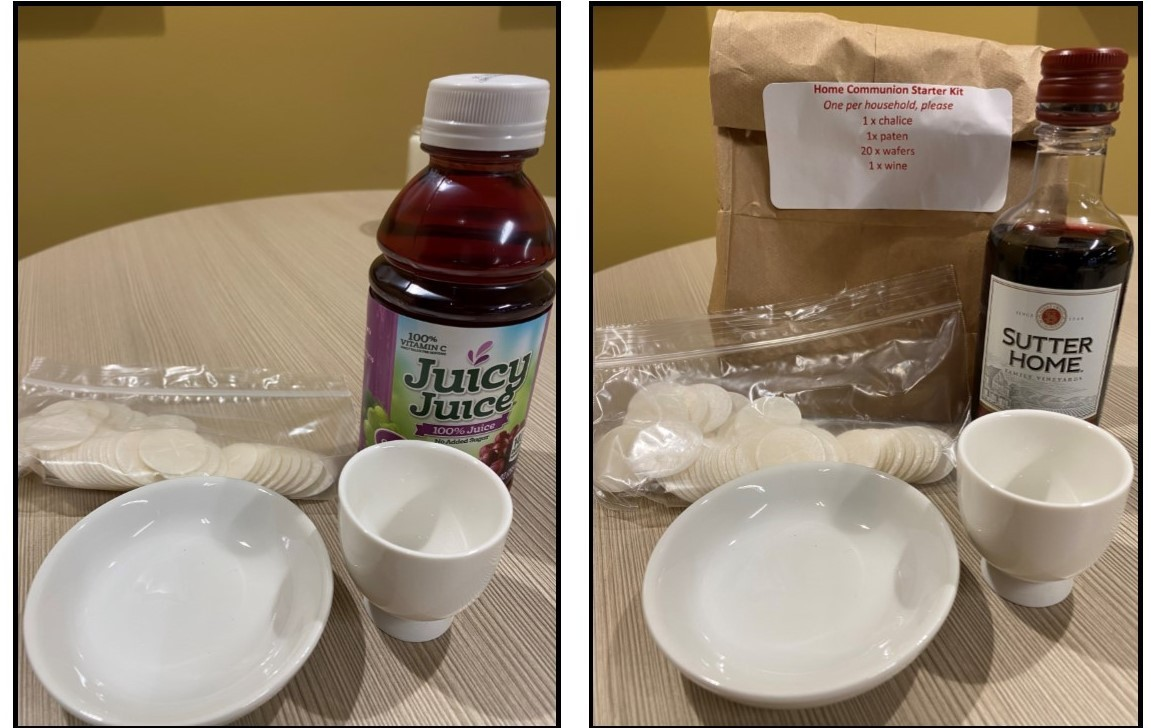table div table+table+table+table+table+table+table+table+table+table+table+table+table+table+table+table+table+table div table{width:100%;padding:0}table div table+table+table+table+table+table+table+table+table+table+table+table+table+table+table+table+table+table div table img{width:96.23%;padding:0;float:none}table div table+table+table+table+table+table+table+table+table+table+table+table+table+table+table+table+table+table div table td{width:100%;padding:0 1.88% 18px}/* styles *//* styles */ Drive-In Worship Service Sunday, November 22 If you are unable to join us on Sunday morning at St. Mark in the parking lot at 9:30 am, the service will be available via Livestream on our YouTube channel.
 table div table+table+table+table+table+table+table+table+table+table+table+table+table+table+table+table+table+table+table+table div table{width:100%;padding:0}table div table+table+table+table+table+table+table+table+table+table+table+table+table+table+table+table+table+table+table+table div table img{width:96.23%;padding:0;float:none}table div table+table+table+table+table+table+table+table+table+table+table+table+table+table+table+table+table+table+table+table div table td{width:100%;padding:0 1.88% 18px}/* styles */Don't forget to turn in your planned for giving cards
On October 18, we announced our 2021 Pledged Giving goal of \$750,000, which is similar to our budget for 2020. To date, we have received 177 Pledges totaling \$542,562. This amount represents 72% of the pledged giving needed to meet our budget goals for the coming year. This year’s stewardship campaign will continue each week until our goal is reached. We thank you for your participation in this important expression of support and gratitude. Together, we can keep our ministry moving forward with confidence and hope.

 /* styles */ If you still need to submit a 2021 planned for giving card, you may do so by clicking this link.
 table div table+table+table+table+table+table+table+table+table+table+table+table+table+table+table+table+table+table+table+table+table+table+table div table{width:100%;padding:0}table div table+table+table+table+table+table+table+table+table+table+table+table+table+table+table+table+table+table+table+table+table+table+table div table img{width:96.23%;padding:0;float:none}table div table+table+table+table+table+table+table+table+table+table+table+table+table+table+table+table+table+table+table+table+table+table+table div table td{width:100%;padding:0 1.88% 18px}/* styles *//* styles */ Blood Drive St. Mark will be sponsoring a blood drive on Tuesday, November 24 from 1:30 - 7:30 P.M. You can schedule a time by calling Jill Davenport at 815-398-3557 or by clicking on this link. Rock River Valley Blood Center is excited to announce that as of August 31st, all successful units donated are being tested for COVID-19 antibodies. The test has been added as part of our regular testing. This is a COVID-19 antibody test, NOT a test for current viral infection. RRVBC implemented the testing in hopes of finding potential convalescent plasma donors. Donors will be able to access their results 7-10 days after their donation date on the RRVBC portal. If you have any additional questions, please call (815)965-8751. If you have recovered from COVID-19, please notify the blood center staff at registration that you tested positive for COVID-19 and want to donate your plasma. Even if you can't give blood, you can still help by assisting with the drive. Pass along the word and encourage your co-workers, family and friends to make an appointment to donate as well.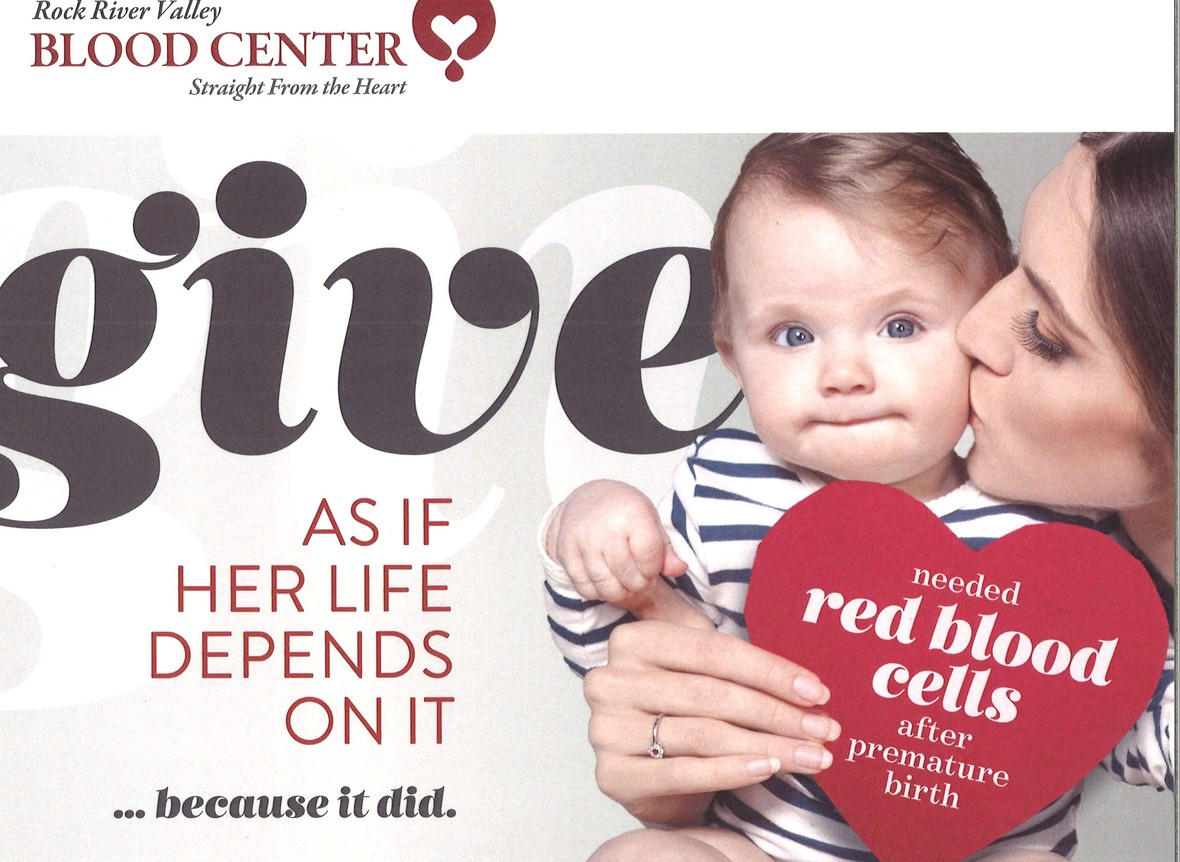table div table+table+table+table+table+table+table+table+table+table+table+table+table+table+table+table+table+table+table+table+table+table+table+table+table+table div table{width:100%;padding:0}table div table+table+table+table+table+table+table+table+table+table+table+table+table+table+table+table+table+table+table+table+table+table+table+table+table+table div table img{width:96.23%;padding:0;float:none}table div table+table+table+table+table+table+table+table+table+table+table+table+table+table+table+table+table+table+table+table+table+table+table+table+table+table div table td{width:100%;padding:0 1.88% 18px}/* styles */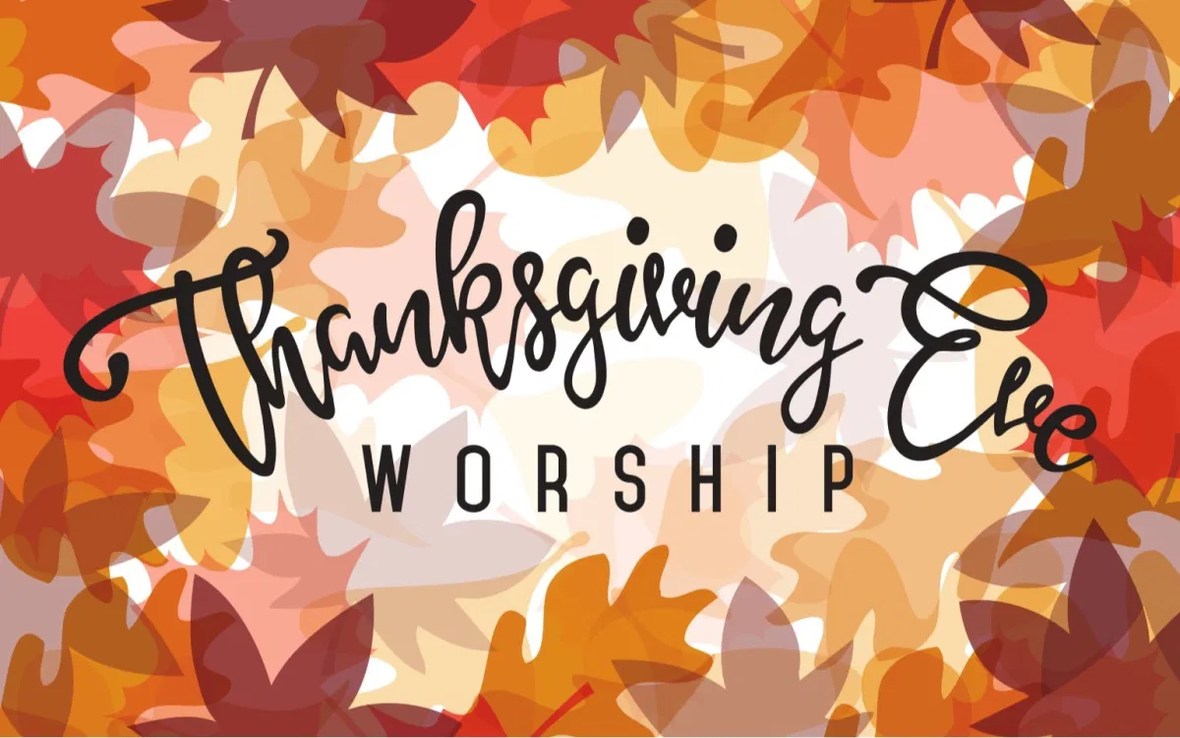/* styles */ Join us for worship on November 25, at 6:30 P.M. for a recorded Thanksgiving worship service on our YouTube channel. We will give thanks and praise for all that the Lord has done!
 table div table+table+table+table+table+table+table+table+table+table+table+table+table+table+table+table+table+table+table+table+table+table+table+table+table+table+table+table+table div table{width:100%;padding:0}table div table+table+table+table+table+table+table+table+table+table+table+table+table+table+table+table+table+table+table+table+table+table+table+table+table+table+table+table+table div table img{width:96.23%;padding:0;float:none}table div table+table+table+table+table+table+table+table+table+table+table+table+table+table+table+table+table+table+table+table+table+table+table+table+table+table+table+table+table div table td{width:100%;padding:0 1.88% 18px}/* styles */This year’s devotional booklet is Heavenly Peace: Daily Prayers for Advent by Sarah Thomas-Tracy. Booklets will be distributed at the November 22 outdoor worship service. Alternatively, you may come to the church to pick up your copy any time during regular office hours, Monday - Friday 9:00 am - 1:00 pm.

 table div table+table+table+table+table+table+table+table+table+table+table+table+table+table+table+table+table+table+table+table+table+table+table+table+table+table+table+table+table+table+table div table{width:100%;padding:0}table div table+table+table+table+table+table+table+table+table+table+table+table+table+table+table+table+table+table+table+table+table+table+table+table+table+table+table+table+table+table+table div table img{width:96.23%;padding:0;float:none}table div table+table+table+table+table+table+table+table+table+table+table+table+table+table+table+table+table+table+table+table+table+table+table+table+table+table+table+table+table+table+table div table td{width:100%;padding:0 1.88% 18px}/* styles */St. Mark Mobile App!

This is different than our church directory app.
Our mobile app is designed specifically for St. Mark to improve our communication with our congregation and nurture our members. We encourage you to download the app. It's about getting involved and staying connected.

 table div table+table+table+table+table+table+table+table+table+table+table+table+table+table+table+table+table+table+table+table+table+table+table+table+table+table+table+table+table+table+table+table+table div table{width:100%;padding:0}table div table+table+table+table+table+table+table+table+table+table+table+table+table+table+table+table+table+table+table+table+table+table+table+table+table+table+table+table+table+table+table+table+table div table img{width:96.23%;padding:0;float:none}table div table+table+table+table+table+table+table+table+table+table+table+table+table+table+table+table+table+table+table+table+table+table+table+table+table+table+table+table+table+table+table+table+table div table td{width:100%;padding:0 1.88% 18px}/* styles */Poinsettias
Our Sanctuary will soon be decorated for Christmas and our poinsettias add a special touch. We will be recording our Christmas concert and the Christmas Eve worship and would like to have our Sanctuary looking festive. The price again this year is \$10.00 per plant.

You may pick up your purchased poinsettias on Monday, December 21, and the days right before Christmas during office hours.

Click on this link to order yours today.

If you would like to stop by the office and fill out a form and pay, you can do that during office hours, Monday - Friday 9:00 a.m. - 1:00 p.m.

 table div table+table+table+table+table+table+table+table+table+table+table+table+table+table+table+table+table+table+table+table+table+table+table+table+table+table+table+table+table+table+table+table+table+table+table div table{width:100%;padding:0}table div table+table+table+table+table+table+table+table+table+table+table+table+table+table+table+table+table+table+table+table+table+table+table+table+table+table+table+table+table+table+table+table+table+table+table div table img{width:96.23%;padding:0;float:none}table div table+table+table+table+table+table+table+table+table+table+table+table+table+table+table+table+table+table+table+table+table+table+table+table+table+table+table+table+table+table+table+table+table+table+table div table td{width:100%;padding:0 1.88% 18px}/* styles */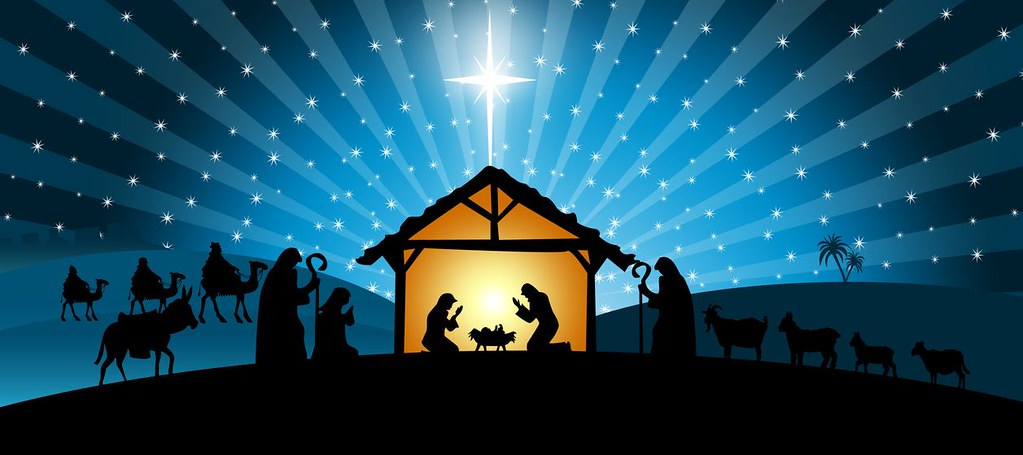SAVE the DATE!
Saturday, December 19th. His Name Is Jesus, Live Drive-Thru Nativity 6:00-9:00 P.M. There will be live animals, characters in costumes, the manger, the story of Jesus birth. There will even be “Wassail and Cookies!” All of this from the warmth of your car. Please consider helping make cookies at home. We are looking for people that enjoy baking to assemble six cookies in a bag or cellophane. Have fun with this! Please e-mail let us know if you will be one of our cookie bakers and how many bags you will make. E-mail Melinda@stmarklc.com for more information or call 815-871-0390.

 table div table+table+table+table+table+table+table+table+table+table+table+table+table+table+table+table+table+table+table+table+table+table+table+table+table+table+table+table+table+table+table+table+table+table+table+table+table div table{width:100%;padding:0}table div table+table+table+table+table+table+table+table+table+table+table+table+table+table+table+table+table+table+table+table+table+table+table+table+table+table+table+table+table+table+table+table+table+table+table+table+table div table img{width:96.23%;padding:0;float:none}table div table+table+table+table+table+table+table+table+table+table+table+table+table+table+table+table+table+table+table+table+table+table+table+table+table+table+table+table+table+table+table+table+table+table+table+table+table div table td{width:100%;padding:0 1.88% 18px}/* styles */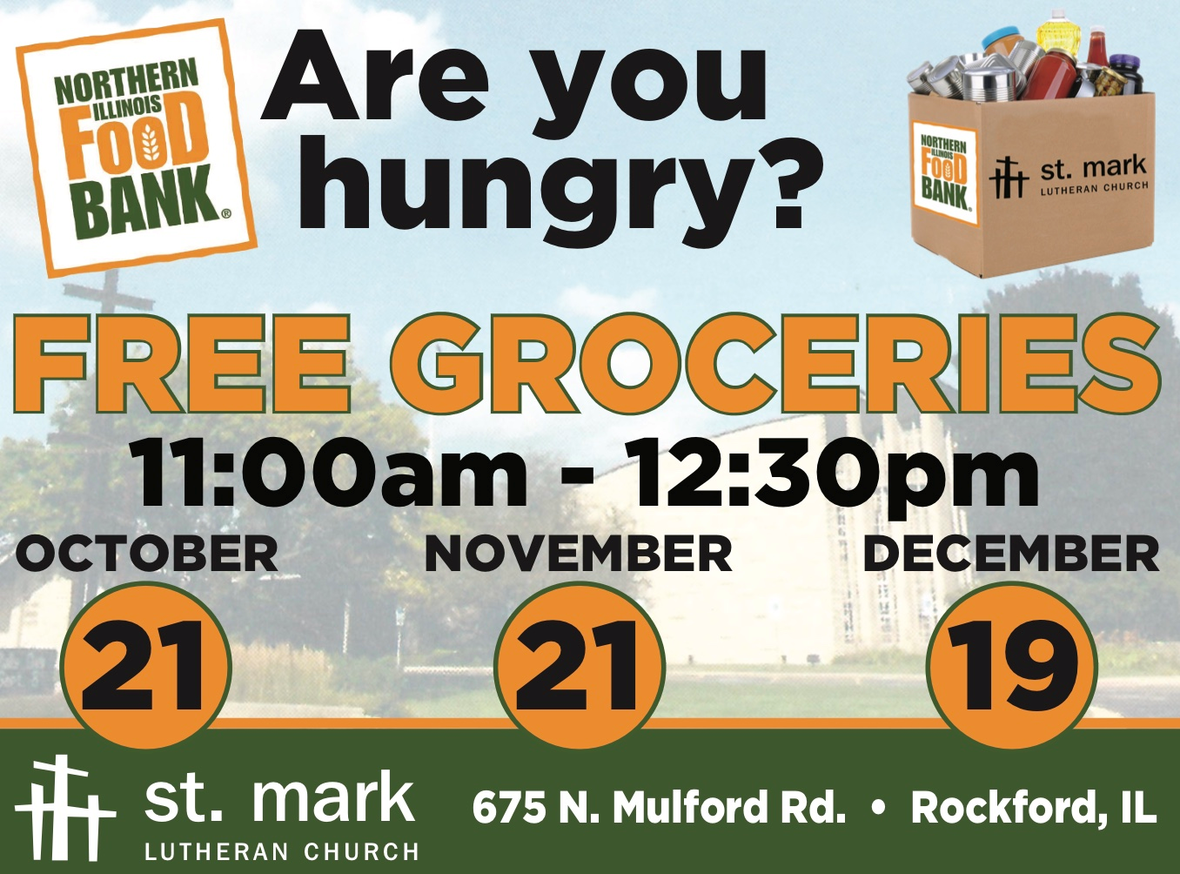table div table+table+table+table+table+table+table+table+table+table+table+table+table+table+table+table+table+table+table+table+table+table+table+table+table+table+table+table+table+table+table+table+table+table+table+table+table+table+table div table{width:100%;padding:0}table div table+table+table+table+table+table+table+table+table+table+table+table+table+table+table+table+table+table+table+table+table+table+table+table+table+table+table+table+table+table+table+table+table+table+table+table+table+table+table div table img{width:96.23%;padding:0;float:none}table div table+table+table+table+table+table+table+table+table+table+table+table+table+table+table+table+table+table+table+table+table+table+table+table+table+table+table+table+table+table+table+table+table+table+table+table+table+table+table div table td{width:100%;padding:0 1.88% 18px}/* styles */Hungry?
Do you know someone that could use some groceries?

We look forward to another successful Mobile Pantry/grocery giveaway on Saturday, November 21. We will be loading groceries into people’s trunks from 11:00-12:30 P.M. If you know someone who needs groceries but cannot drive to the church please consider picking up groceries for them, or call 815-871-0390 and we will have a volunteer deliver to them. “Many hands make light work.”

Thank you to our list of volunteers who signed up. We will see you at 10:30 A.M. dressed for the weather, with a mask and gloves, please.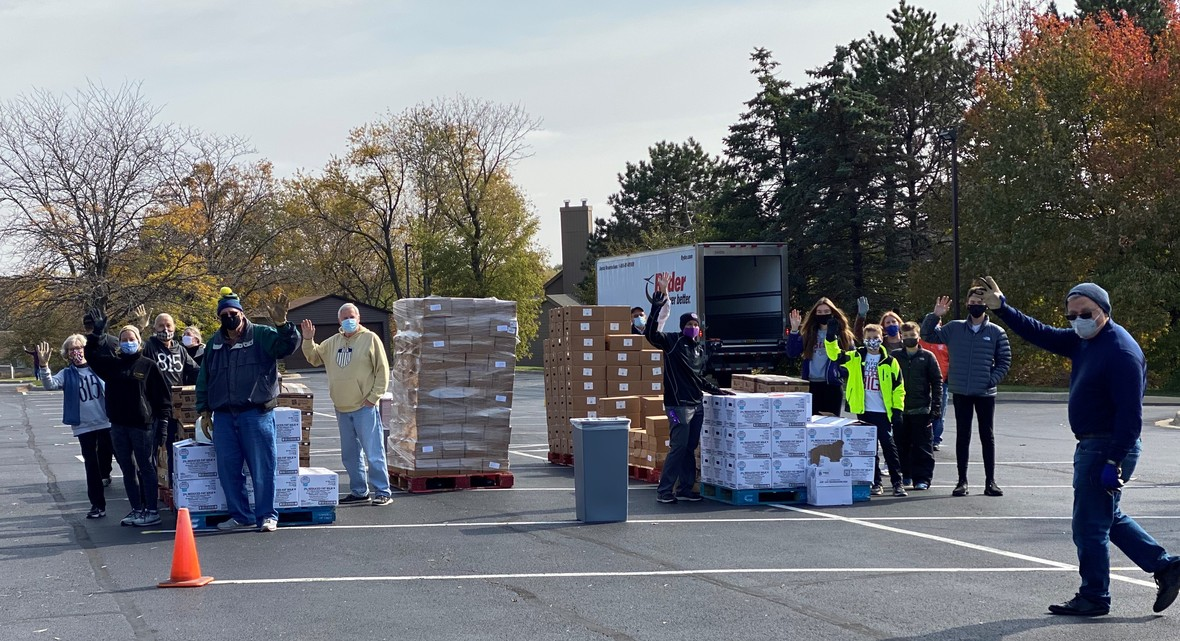table div table+table+table+table+table+table+table+table+table+table+table+table+table+table+table+table+table+table+table+table+table+table+table+table+table+table+table+table+table+table+table+table+table+table+table+table+table+table+table+table+table+table div table{width:100%;padding:0}table div table+table+table+table+table+table+table+table+table+table+table+table+table+table+table+table+table+table+table+table+table+table+table+table+table+table+table+table+table+table+table+table+table+table+table+table+table+table+table+table+table+table div table img{width:96.23%;padding:0;float:none}table div table+table+table+table+table+table+table+table+table+table+table+table+table+table+table+table+table+table+table+table+table+table+table+table+table+table+table+table+table+table+table+table+table+table+table+table+table+table+table+table+table+table div table td{width:100%;padding:0 1.88% 18px}/* styles */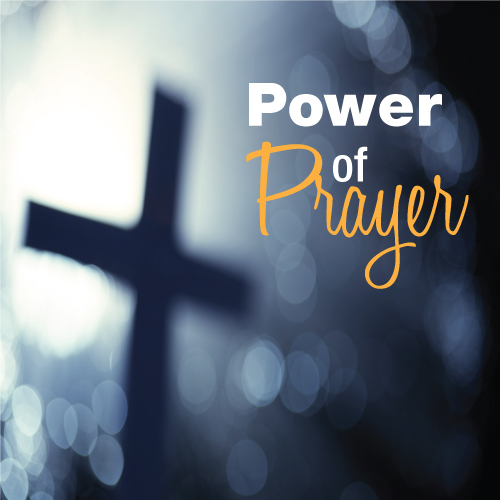St. Mark Prayer Chain
Would you like to be a part of the St. Mark prayer chain? Prayer requests come into the church office weekly. As we receive prayer requests we would send out an e-mail and ask that you pray daily for the persons mentioned in the requests. All requests are to remain confidential. Please e-mail melinda@stmarklc.com if you would like to participate in the prayer chain. “Be anxious for nothing, but in everything by prayer and supplication, with thanksgiving, let your requests be made known to God.” Colossians 4:2.

 table div table+table+table+table+table+table+table+table+table+table+table+table+table+table+table+table+table+table+table+table+table+table+table+table+table+table+table+table+table+table+table+table+table+table+table+table+table+table+table+table+table+table+table+table div table{width:100%;padding:0}table div table+table+table+table+table+table+table+table+table+table+table+table+table+table+table+table+table+table+table+table+table+table+table+table+table+table+table+table+table+table+table+table+table+table+table+table+table+table+table+table+table+table+table+table div table img{width:96.23%;padding:0;float:none}table div table+table+table+table+table+table+table+table+table+table+table+table+table+table+table+table+table+table+table+table+table+table+table+table+table+table+table+table+table+table+table+table+table+table+table+table+table+table+table+table+table+table+table+table div table td{width:100%;padding:0 1.88% 18px}/* styles */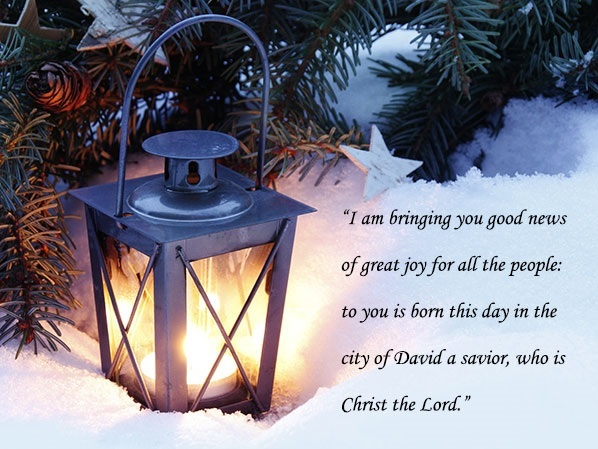Volunteer Opportunities in December
Youth Ministry is looking for six families to be Luminary Families. We need your help on Saturday, December 19, at 5:00 P.M. for an hour. We also need help on December 24th for one hour. Please sign-up for one or both dates. Please e-mail melinda@stmarklc.com for more information and to sign-up.

Are you looking to volunteer? The Northern Illinois Food Bank does a good job of social distancing and following guidelines during the assembly lines for loading produce etc into boxes. If you want to find out more about the food bank, go to www.solvehungertoday.org. Click on the volunteer link to see the calendar. There are opportunities Monday through Saturday. If you would be interested in doing this as a group please e-mail melinda@stmarklc.com.

 table div table+table+table+table+table+table+table+table+table+table+table+table+table+table+table+table+table+table+table+table+table+table+table+table+table+table+table+table+table+table+table+table+table+table+table+table+table+table+table+table+table+table+table+table+table+table div table{width:100%;padding:0}table div table+table+table+table+table+table+table+table+table+table+table+table+table+table+table+table+table+table+table+table+table+table+table+table+table+table+table+table+table+table+table+table+table+table+table+table+table+table+table+table+table+table+table+table+table+table div table img{width:96.23%;padding:0;float:none}table div table+table+table+table+table+table+table+table+table+table+table+table+table+table+table+table+table+table+table+table+table+table+table+table+table+table+table+table+table+table+table+table+table+table+table+table+table+table+table+table+table+table+table+table+table+table div table td{width:100%;padding:0 1.88% 18px}/* styles */Instant Church Directory
If you are one of many that have downloaded the mobile app or are using the church directory on your computer, you know what a great tool this is. It is always current and up to date.

Please take a moment to download the app or create an account on your computer. The directions are listed below, but if you are having trouble or would just like some help, please call Jill Davenport at 815-398-3557.

Also, many of you have not submitted photos. To do that, follow the link below or email your photo to Jill@stmarklc.com

Instant Church Directory

Mobile App Login Instructions for Android & Kindle Devices

We also have a form for you to complete to make sure we have the most current information for the directory. There are paper forms at church or click on the link to Church Directory Form

 table div table+table+table+table+table+table+table+table+table+table+table+table+table+table+table+table+table+table+table+table+table+table+table+table+table+table+table+table+table+table+table+table+table+table+table+table+table+table+table+table+table+table+table+table+table+table+table+table div table{width:100%;padding:0}table div table+table+table+table+table+table+table+table+table+table+table+table+table+table+table+table+table+table+table+table+table+table+table+table+table+table+table+table+table+table+table+table+table+table+table+table+table+table+table+table+table+table+table+table+table+table+table+table div table img{width:96.23%;padding:0;float:none}table div table+table+table+table+table+table+table+table+table+table+table+table+table+table+table+table+table+table+table+table+table+table+table+table+table+table+table+table+table+table+table+table+table+table+table+table+table+table+table+table+table+table+table+table+table+table+table+table div table td{width:100%;padding:0 1.88% 18px}/* styles */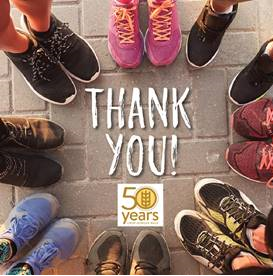CROP Hunger Walk was a Success!
It has been five years since the Rockford Area CROP Hunger Walk raised so much money. Thanks to the hard work of 27 organizations over \$38,000 have been raised to fight hunger here and around the world.

Because of last year’s wonderful effort an ambitious goal of \$35,000 was set for 2020. Covid-19 made this a special challenge and people responded marvelously well. The pandemic continues to increase worldwide hunger as never before. Your response to this fact has been most heart warm.

 table div table+table+table+table+table+table+table+table+table+table+table+table+table+table+table+table+table+table+table+table+table+table+table+table+table+table+table+table+table+table+table+table+table+table+table+table+table+table+table+table+table+table+table+table+table+table+table+table+table+table div table{width:100%;padding:0}table div table+table+table+table+table+table+table+table+table+table+table+table+table+table+table+table+table+table+table+table+table+table+table+table+table+table+table+table+table+table+table+table+table+table+table+table+table+table+table+table+table+table+table+table+table+table+table+table+table+table div table img{width:96.23%;padding:0;float:none}table div table+table+table+table+table+table+table+table+table+table+table+table+table+table+table+table+table+table+table+table+table+table+table+table+table+table+table+table+table+table+table+table+table+table+table+table+table+table+table+table+table+table+table+table+table+table+table+table+table+table div table td{width:100%;padding:0 1.88% 18px}/* styles */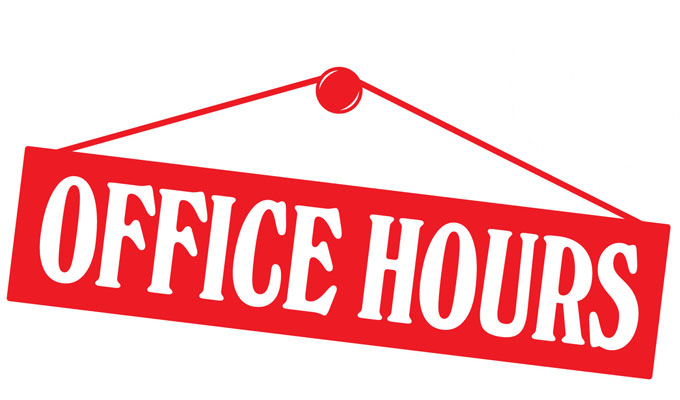Office Hours
Monday through Friday 9:00 A.M. - 1:00 P.M. As always, please call the office or Pastor Chad if you have any needs or concerns.

 table div table+table+table+table+table+table+table+table+table+table+table+table+table+table+table+table+table+table+table+table+table+table+table+table+table+table+table+table+table+table+table+table+table+table+table+table+table+table+table+table+table+table+table+table+table+table+table+table+table+table+table+table div table{width:100%;padding:0}table div table+table+table+table+table+table+table+table+table+table+table+table+table+table+table+table+table+table+table+table+table+table+table+table+table+table+table+table+table+table+table+table+table+table+table+table+table+table+table+table+table+table+table+table+table+table+table+table+table+table+table+table div table img{width:96.23%;padding:0;float:none}table div table+table+table+table+table+table+table+table+table+table+table+table+table+table+table+table+table+table+table+table+table+table+table+table+table+table+table+table+table+table+table+table+table+table+table+table+table+table+table+table+table+table+table+table+table+table+table+table+table+table+table+table div table td{width:100%;padding:0 1.88% 18px}/* styles */## Outreach Ministry

 table div table+table+table+table+table+table+table+table+table+table+table+table+table+table+table+table+table+table+table+table+table+table+table+table+table+table+table+table+table+table+table+table+table+table+table+table+table+table+table+table+table+table+table+table+table+table+table+table+table+table+table+table+table+table div table{width:100%;padding:0}table div table+table+table+table+table+table+table+table+table+table+table+table+table+table+table+table+table+table+table+table+table+table+table+table+table+table+table+table+table+table+table+table+table+table+table+table+table+table+table+table+table+table+table+table+table+table+table+table+table+table+table+table+table+table div table img{width:96.23%;padding:0;float:none}table div table+table+table+table+table+table+table+table+table+table+table+table+table+table+table+table+table+table+table+table+table+table+table+table+table+table+table+table+table+table+table+table+table+table+table+table+table+table+table+table+table+table+table+table+table+table+table+table+table+table+table+table+table+table div table td{width:100%;padding:0 1.88% 18px}/* styles */Foster Care Christmas Collection is November 30
Please remember these children who are served by Lutheran Social Services. They have requested gift cards, \$25 or \$30, or cash donations this year due to the virus pandemic.

You may send gift cards or checks to St. Mark or donate online at our St. Mark website. Note on each donation your gifts are to be dedicated to our area foster care children.

Help make Christmas happy for these kids. The Outreach Committee thanks you for your great generosity.

 table div table+table+table+table+table+table+table+table+table+table+table+table+table+table+table+table+table+table+table+table+table+table+table+table+table+table+table+table+table+table+table+table+table+table+table+table+table+table+table+table+table+table+table+table+table+table+table+table+table+table+table+table+table+table+table+table div table{width:100%;padding:0}table div table+table+table+table+table+table+table+table+table+table+table+table+table+table+table+table+table+table+table+table+table+table+table+table+table+table+table+table+table+table+table+table+table+table+table+table+table+table+table+table+table+table+table+table+table+table+table+table+table+table+table+table+table+table+table+table div table img{width:96.23%;padding:0;float:none}table div table+table+table+table+table+table+table+table+table+table+table+table+table+table+table+table+table+table+table+table+table+table+table+table+table+table+table+table+table+table+table+table+table+table+table+table+table+table+table+table+table+table+table+table+table+table+table+table+table+table+table+table+table+table+table+table div table td{width:100%;padding:0 1.88% 18px}/* styles */## Our St. Mark members:

Jan Cotter, Dennis Howe, John Johnson, Roger Lind, Pat Venstrom, Jim Tooley, Diane Swanson, Nancy Scheel, Billie Reinhold, Constance Carlson, Sherri Lindquist, Tim Vivian, Bill Jennings, Tim Panjkovich, Bette Patterson, Jerry Cazaniga, David Young, Keith Haggestad.

## Our condolences to:

the family and long time friend, Dixie Simons of James Stewart, upon his death on Saturday, November 14.

Betty Lundvall upon the death of her sister, Ruth Zahler.

## Family and friends of St. Mark members:

Dana Long, Butch Cotter, Wanda Adamson, Milane Bagel, Gayle Coffler, Darold Beekmann, Janet Webster, Rae Ann McIntire, Linda Johnson, Robert and Cindy Wilson, Pastor Jane McChesney, Emily Tropp, Katelynn Audette, Eva Love Sherbondy, Norma Comstock, Carolyn King, Ashton Henley, Nathan Gyllin.

## Those serving in the military and their families:

Hunter Haggestad, Tristan Davenport, Scot Hornick, Bailey Panjkovich, Xander Gehrke, Jim Tammen, Brandon Ballenger, Taylor Eversole, Dean Barron.

Strengthen and encourage those in public health services and in the medical profession: care-givers, nurses, attendants, doctors, all who commit themselves to care for the sick and their families.

 table div table+table+table+table+table+table+table+table+table+table+table+table+table+table+table+table+table+table+table+table+table+table+table+table+table+table+table+table+table+table+table+table+table+table+table+table+table+table+table+table+table+table+table+table+table+table+table+table+table+table+table+table+table+table+table+table+table+table+table div table{width:100%;padding:0}table div table+table+table+table+table+table+table+table+table+table+table+table+table+table+table+table+table+table+table+table+table+table+table+table+table+table+table+table+table+table+table+table+table+table+table+table+table+table+table+table+table+table+table+table+table+table+table+table+table+table+table+table+table+table+table+table+table+table+table div table img{width:96.23%;padding:0;float:none}table div table+table+table+table+table+table+table+table+table+table+table+table+table+table+table+table+table+table+table+table+table+table+table+table+table+table+table+table+table+table+table+table+table+table+table+table+table+table+table+table+table+table+table+table+table+table+table+table+table+table+table+table+table+table+table+table+table+table+table div table td{width:100%;padding:0 1.88% 18px}/* styles *//* styles */ Office Hours Monday through Friday 9:00 A.M. - 1:00 P.M. Telephone: 815-398-3557 Publications St. Mark Memos, the weekly e-newsletter, is distributed each Thursday. The deadline for submission is Monday by 3:00 P.M. Please click to send submissions. Sermons Online Miss a Sunday at St. Mark? Please visit our website and watch the sermons online. Please click to watch.